### Home > CALC > Chapter 5 > Lesson 5.1.3 > Problem5-32

5-32.
1. Examine the integrals below. Consider the multiple tools available for evaluating integrals and use the best strategy for each. After evaluating the integral, write a short description of your method. Homework Help ✎

1.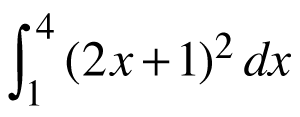2.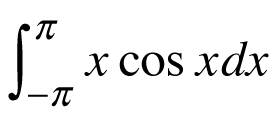3.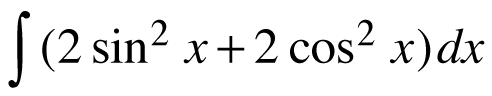4.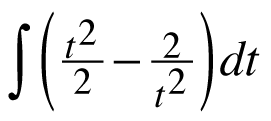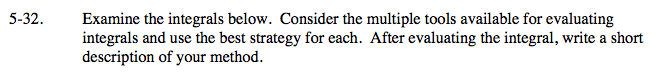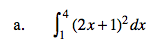Expand the integrand first.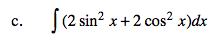Factor and use a trigonometry identity.

$\int 2(\text{sin}^{2}x+\text{cos}^{2}x)dx=\int 2dx= 2x+C$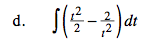How could you simplify so you can use the Power Rule?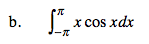Can you calculate the integral just by looking at the graph?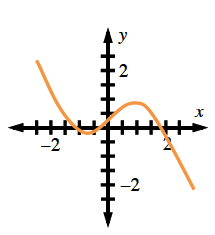Without graphing, how could you have recognized that the integrand is odd?